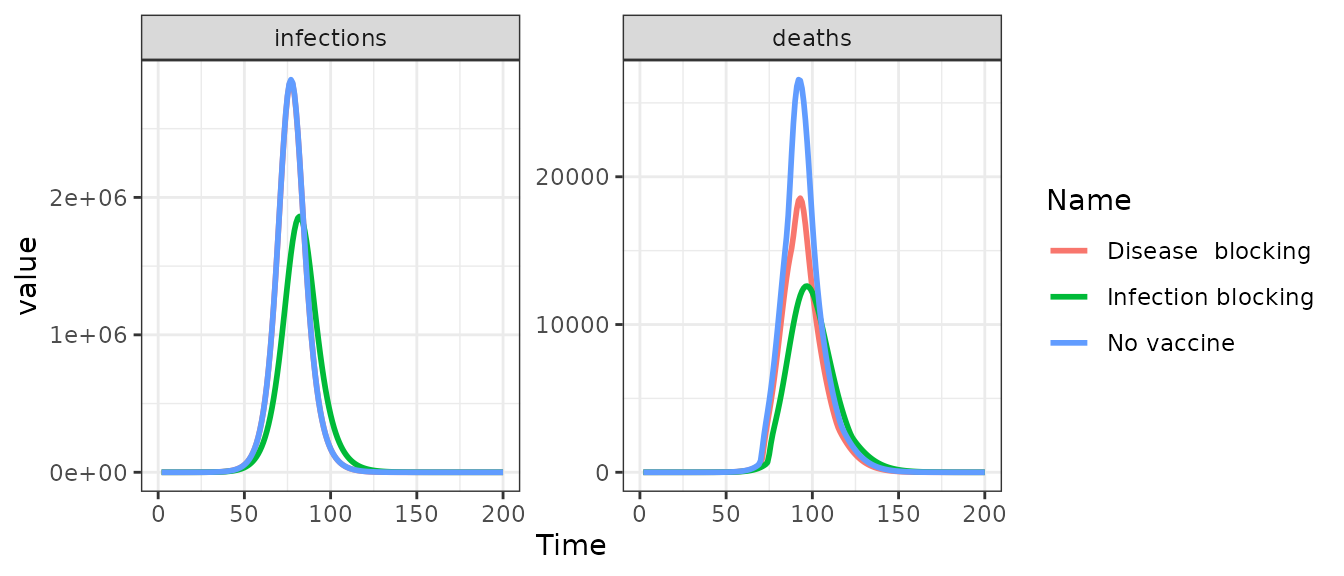library(nimue)
library(ggplot2)
library(dplyr)

We can parameterise the mode of action of the vaccine to be infection-blocking, anti-disease or both.

### No vaccine

First, let’s start with a counterfactual run with no vaccination:

# Run the model with an example population and no vaccination
no_vaccine <- run(country = "United Kingdom",
max_vaccine = 0)
# Format the output selecting infection and deaths
out1 <- format(no_vaccine, compartments = NULL, summaries = c("infections", "deaths")) %>%
mutate(Name = "No vaccine")

### Infection-blocking vaccine

Next, we can run with an infection-blocking vaccine:

# Run the determinstic model with an example population and infection-blocking vaccine
infection_blocking <- run(country = "United Kingdom",
max_vaccine = 200000,
vaccine_efficacy_disease = rep(0, 17),
vaccine_efficacy_infection = rep(0.9, 17))
# Format the output selecting infection and deaths
out2 <- format(infection_blocking, compartments = NULL, summaries = c("infections", "deaths")) %>%
mutate(Name = "Infection blocking")

### Anti-disease vaccine

And finally, a run with a disease-blocking vaccine:

# Run the determinstic model with an example population and anti-disease vaccine
disease_blocking <- run(country = "United Kingdom",
max_vaccine = 200000,
vaccine_efficacy_disease = rep(0.9, 17),
vaccine_efficacy_infection = rep(0, 17))
# Format the output selecting infection and deaths
out3 <- format(disease_blocking, compartments = NULL, summaries = c("infections", "deaths")) %>%
mutate(Name = "Disease  blocking")

### Compare

# Create plot data.frame
pd <- bind_rows(out1, out2, out3)
# Plot outputs
ggplot(pd, aes(x = t, y = value, group = Name, col = Name)) +
geom_line(size = 1) +
facet_wrap(~ compartment, scales = "free_y", ncol = 2) +
xlim(0, 200) +
xlab("Time") +
theme_bw()
#> Warning: Removed 498 row(s) containing missing values (geom_path).### Multiple properties

It is possible to parameterise the vaccine to be both infection blocking and disease blocking. If doing so, be careful as these efficacies compound (blocking an infection, by definition, also blocks any downstream consequences).